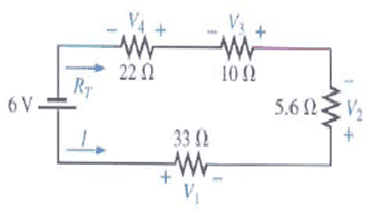# Minimum wattage rating ?

Lord Dark
Minimum wattage rating ??

## Homework Statement

Hi everyone ,,

got the following questiona. Find the total resistance, current and unknown voltage drops
b. Verify KVL around closed loop
c. Find the power dissipated by each resistor and note whether the power delivered is equal to the dissipated
d. If the resistors available with wattage rating of ½ , 1 and 2 W, what is the minimum wattage rating can be used for each resistance.

## The Attempt at a Solution

I've solved them all except d ,, I didn't understand what question d actually means ...
can someone explain and answer for me for one resistor so I can get the others please :)

#### Attachments

Homework Helper
d. If the resistors available with wattage rating of ½ , 1 and 2 W, what is the minimum wattage rating can be used for each resistance.

I've solved them all except d ,, I didn't understand what question d actually means ...
can someone explain and answer for me for one resistor so I can get the others please :)

Hi Lord Dark!I think it means that the resistor burns out if it carries more than the rated wattage, so calculate the watts through each resistor and choose the next rate up.Lord Dark

Hi tiny-timI think it means that the resistor burns out if it carries more than the rated wattage, so calculate the watts through each resistor and choose the next rate up.

by saying choose the next rate up meaning ??1.calculate the watts in the resistor then choose the watts of the next one ?

example : r1=0.24 w & r2=0.04 w ,, choose the minimum rate for r1 is 0.04??

or

2.just get their power & choose the higher one ?

example : r1=0.24 w so minimum wattage = 0.5 ,, r2=0.04 w MW = 0.5 too ??
(then all of them the minimum will be 0.5 w because they all are less then 0.5)

and BTW why is the question saying (the resistors available with wattage rating of 0.5,1 and 2 W) the maximum wattage I got is in resistor 1 which is 0.24 w .. so why is it saying 2 w and 1 w ?? :grumpy:

Homework Helper
2.just get their power & choose the higher one ?

example : r1=0.24 w so minimum wattage = 0.5 ,, r2=0.04 w MW = 0.5 too ??
(then all of them the minimum will be 0.5 w because they all are less then 0.5)

and BTW why is the question saying (the resistors available with wattage rating of 0.5,1 and 2 W) the maximum wattage I got is in resistor 1 which is 0.24 w .. so why is it saying 2 w and 1 w ?? :grumpy:

Hi Lord Dark!I think it's because this isn't a maths world, in which you can go into a store and but a 0.24W resistor …

in the real world, you can only buy 0.5W 1W 2W etc …

in this case you buy all 0.5WLord Dark

ha ha,, thanks tiny-tim - again - ,, still didn't work in a lab ~.~